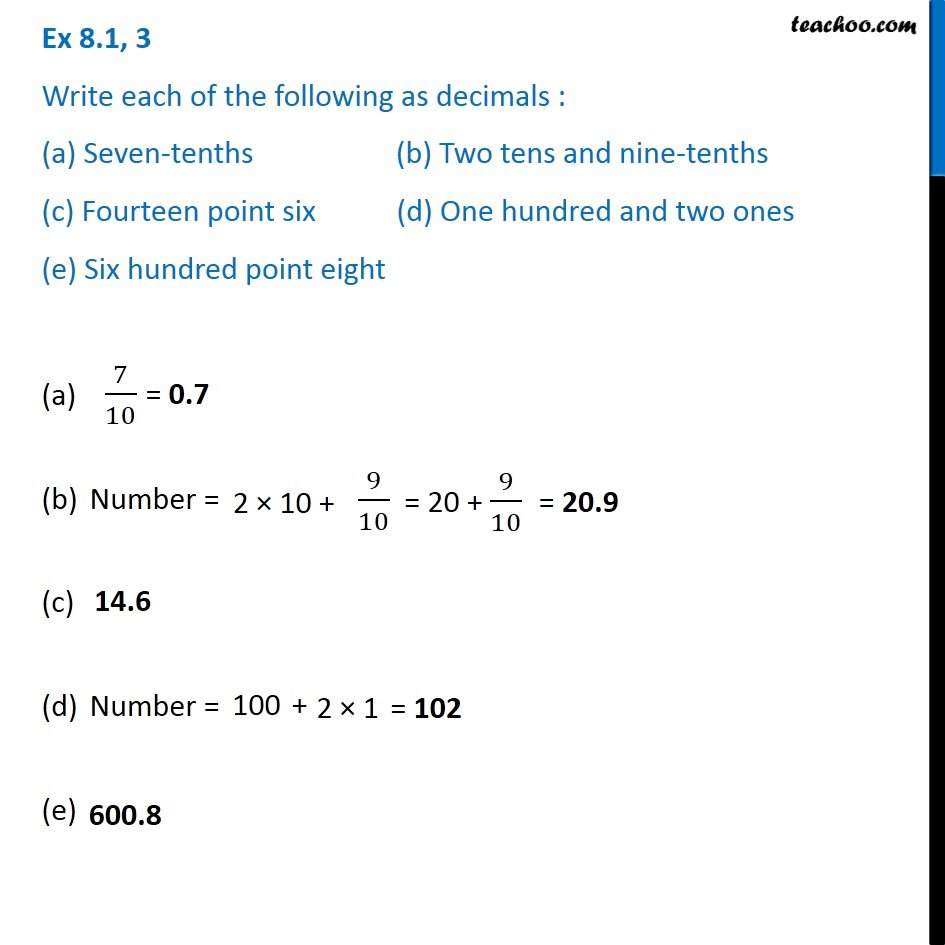1. Chapter 8 Class 6 Decimals
2. Concept wise
3. Words to decimals

Transcript

Ex 8.1, 3 Write each of the following as decimals : (a) Seven-tenths (b) Two tens and nine-tenths (c) Fourteen point six (d) One hundred and two ones (e) Six hundred point eight Number = Number = (e)